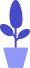# Python define variables

Estimated reading: 3 minutes 116 views

A variable is like a box for storing things. We put the things we want to store in this box, and then give the box a name. When we need to use the contents of the box, we just need to say the name of the box and we can find the contents.

The contents of the box can be changed, that is, we can take out the original things in the box and put other things in.

For example, let’s name this box (variable) box and put the number 12 in it. Then, you can use box to refer to this variable later, and its value is 12. When we take 12 out of the box and put another number 21, if we refer to the variable box again, its value becomes 21, as shown in Figure 1.

In Python, declaring a variable is as simple as giving the variable a name and `=`assigning it a value with an equals sign. This equal sign is called the assignment operator, the `=`left side of the assignment operator is a variable name, and the right side of the assignment operator `=`is the value stored in the variable. Variables can store integers, decimals, or characters, etc.

For example, we declare a variable called box and assign 12 to the variable box.

```				```
>>> box=12
```
```

Then, we can type box after the prompt to see what’s in this variable.

```				```
>>> box
12
```
```

We see that the content in the box is 12. If we reassign the number 21 to the box, the value of the box changes from 12 to 21, which is equivalent to the operation shown in Figure 2-1.

```				```
>>> box=21
>>> box
21
```
```

## Multiple variable assignments

We can also use a single statement to assign values ​​to multiple variables at the same time, for example, we can set the variables a, b, and c to 1.

```				```
>>> a=b=c=1
```
```

This is called multivariable assignment.

Now, we can see that the variables a, b and c are now all equal to 1.

```				```
>>> a
1
>>> b
1
>>> c
1
```
```

## Incremental assignment

In Python 3, the equal sign can be combined with an arithmetic operator to reassign the result of the calculation to the variable on the left. This is called increment assignment.

```				```
>>> age=9+1
>>> age
10
```
```